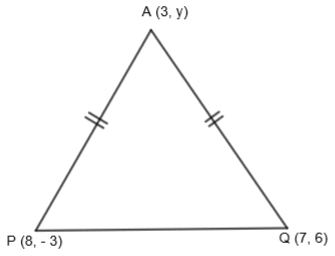QuestionAnswers

# If A (3, y) is equidistant from points P (8, – 3) and Q (7, 6), find the value of y and find the distance AQ.Hint: Equate the distances AP and AQ by using the distance formula to find the distance between any two points $\left( {{x}_{1}},{{y}_{1}} \right)\text{ and }\left( {{x}_{2}},{{y}_{2}} \right)$ which is $\sqrt{{{\left( {{x}_{2}}-{{x}_{1}} \right)}^{2}}+{{\left( {{y}_{2}}-{{y}_{1}} \right)}^{2}}}$. From this find the value of y. Now substitute the value of y in distance AQ to find the value of AQ.
We are given that if A (3, y) is equidistant from points P (8, – 3) and Q (7, 6), then we have to find the value of y and AQ.We know the distance between any two points $M\left( {{x}_{1}},{{y}_{1}} \right)\text{ and }N\left( {{x}_{2}},{{y}_{2}} \right)$ can be found by using the distance formula which is
$MN=\sqrt{{{\left( {{x}_{2}}-{{x}_{1}} \right)}^{2}}+{{\left( {{y}_{2}}-{{y}_{1}} \right)}^{2}}}$
So, by using distance formula, we get the distance between points A (3, y) and P (8, – 3) as
$AP=\sqrt{{{\left( 8-3 \right)}^{2}}+{{\left( -3-y \right)}^{2}}}$
$AP=\sqrt{{{\left( 5 \right)}^{2}}+{{\left( -\left( y+3 \right) \right)}^{2}}}$
$AP=\sqrt{25+{{\left( y+3 \right)}^{2}}}....\left( i \right)$
Again by using distance formula, we get the distance between points A (3, y) and Q (7, 6) as
$AQ=\sqrt{{{\left( 7-3 \right)}^{2}}+{{\left( 6-y \right)}^{2}}}$
$AQ=\sqrt{{{\left( 4 \right)}^{2}}+{{\left( -\left( y-6 \right) \right)}^{2}}}$
$AQ=\sqrt{16+{{\left( y-6 \right)}^{2}}}....\left( ii \right)$
We are given that point A is equidistant from points P and Q. So, we get,
AP = AQ.
By substituting the value of AP and AQ from equation (i) and (ii) respectively, we get,
$\sqrt{25+{{\left( y+3 \right)}^{2}}}=\sqrt{16+{{\left( y-6 \right)}^{2}}}.....\left( iii \right)$
By squaring both sides of the above equation, we get,
$25+{{\left( y+3 \right)}^{2}}=16+{{\left( y-6 \right)}^{2}}$
By using ${{\left( a\pm b \right)}^{2}}={{a}^{2}}+{{b}^{2}}\pm 2ab$, we get,
$25+{{\left( y \right)}^{2}}+{{\left( 3 \right)}^{2}}+2\left( 3 \right)y=16+{{y}^{2}}+{{\left( 6 \right)}^{2}}-2\left( 6 \right)y$
$25+{{y}^{2}}+9+6y=16+{{y}^{2}}+36-12y$
By canceling the like terms and simplifying the above equation, we get,
$25+9+6y=16+36-12y$
$34+6y=52-12y$
$6y+12y=52-34$
$18y=18$
$y=1$
Now, by substituting the value of y = 1 in equation (ii), we get,
$AQ=\sqrt{16+{{\left( 1-6 \right)}^{2}}}$
$AQ=\sqrt{16+{{\left( -5 \right)}^{2}}}$
$AQ=\sqrt{16+25}$
$AQ=\sqrt{41}$
So, we get the value of AQ as $\sqrt{41}$ and y as 1.

Note: In this question, as it is given that A is equidistant from points P and Q, many students make this mistake of considering A as the midpoint of line PQ and get $y=\dfrac{6-3}{2}=\dfrac{3}{2}$ which is wrong because if a point is equidistant from 2 other points, it can or cannot be the midpoint. So, this is not a necessary condition. So, in these types of questions, students are advised to use distance formula only.
Table of 3 - Multiplication Table of 3NCERT Class 8 Social Science - Our Past 3 Book PDFNCERT Class 3 Maths Book PDFCBSE Class 12 Maths Chapter-3 Matrices FormulaIMO Maths Syllabus for Class 3CBSE Class 10 Maths Chapter 3 - Pair of Linear Equations in Two Variables FormulaNCERT Book for Class 8 Social Science Our Past 3 PDF in HindiCBSE Class 8 Maths Chapter 3 - Understanding Quadrilaterals FormulasWhere There is a Will There is a Way EssayImportant Questions for CBSE Class 8 Maths Chapter 3 - Understanding QuadrilateralsImportant Questions for CBSE Class 8 Social Science Our Past 3 Chapter 3 - Ruling the CountrysideImportant Questions for CBSE Class 8 Social Science Our Past 3 Chapter 10 - India After IndependenceImportant Questions for CBSE Class 10 Maths Chapter 3 - Pair of Linear Equations in Two VariablesImportant Questions for CBSE Class 8 Social Science Resources and Development Chapter 3 - Mineral and Power ResourcesImportant Questions for CBSE Class 8 Social Science Our Past 3 Chapter 6 - Weavers, Iron Smelters and Factory OwnersImportant Questions for CBSE Class 6 Maths Chapter 3 - Playing with NumbersImportant Questions for CBSE Class 8 Social Science Our Past 3 Chapter 7 - Civilising the Native, Educating the NationImportant Questions for CBSE Class 8 Social Science - Social and Political Life Chapter 3 - Why do we need a ParliamentImportant Questions for CBSE Class 8 Science Chapter 3 - Synthetic Fibres and PlasticsCBSE Class 10 Hindi A Question Paper 2020Hindi A Class 10 CBSE Question Paper 2009Hindi A Class 10 CBSE Question Paper 2015Hindi A Class 10 CBSE Question Paper 2016Hindi A Class 10 CBSE Question Paper 2012Hindi A Class 10 CBSE Question Paper 2010Hindi A Class 10 CBSE Question Paper 2008Hindi A Class 10 CBSE Question Paper 2014Hindi A Class 10 CBSE Question Paper 2007Hindi A Class 10 CBSE Question Paper 2013RS Aggarwal Solutions Class 10 Chapter 3 - Linear Equations in two variables (Ex 3H) Exercise 3.8RD Sharma Class 10 Solutions Chapter 3 - Exercise 3.8RD Sharma Class 8 Solutions Chapter 3 - Square and Square Roots (Ex 3.6) Exercise 3.6RS Aggarwal Solutions Class 8 Chapter-3 Squares and Square Roots (Ex 3G) Exercise 3.7RD Sharma Class 8 Solutions Chapter 3 - Square and Square Roots (Ex 3.7) Exercise 3.7RS Aggarwal Solutions Class 10 Chapter 3 - Linear Equations in two variables (Ex 3F) Exercise 3.6RS Aggarwal Solutions Class 8 Chapter-3 Squares and Square Roots (Ex 3F) Exercise 3.6RS Aggarwal Solutions Class 10 Chapter 3 - Linear Equations in two variables (Ex 3G) Exercise 3.7RD Sharma Class 10 Solutions Chapter 3 - Exercise 3.6NCERT Solutions for Class 10 Maths Chapter 3 Pair of Linear Equations in Two Variables (Ex 3.6) Exercise 3.6